# Hyperbolic Functions

Also found in: Dictionary, Wikipedia.
Related to Hyperbolic Functions: Hyperbolic cosine, Inverse hyperbolic functions

## hyperbolic functions

[¦hī·pər¦bäl·ik ′fəŋk·shənz]
(mathematics)
The real or complex functions sinh (x), cosh (x), tanh (x), coth (x), sech (x), csch (x); they are related to the hyperbola in somewhat the same fashion as the trigonometric functions are related to the circle, and have properties analogous to those of the trigonometric functions.

## Hyperbolic Functions

functions defined by the equations

sinh x =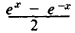(hyperbolic sine of x)

cosh x =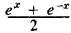(hyperbolic cosine of x)

In some cases, the hyperbolic tangent is also considered:

tanh x =(See Figure 1 for graphs of the hyperbolic functions.) Hyperbolic functions are connected by relations similar to those connecting the trigonometric functions:

cosh2x - sinh2 = 1

tanh x = sinh x/cosh x

sinh (x±y) = sinh x cosh y ± cosh x sinh y

cosh (x±y) = cosh x cosh y ± sinh x sinh y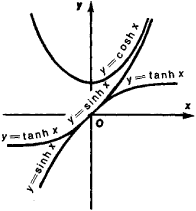Figure 1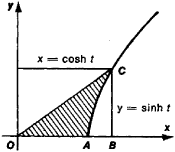Figure 2

Hyperbolic functions may be expressed in terms of trigonometric functions: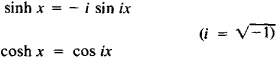Geometrically, the hyperbolic functions are obtained by analysis of the rectangular hyperbola x2 - y2 = 1, which may be defined in terms of the parametric equations x = cosh t and y = sinh t. The argument t represents twice the area of the hyperbolic sector OAC (see Figure 2). Inverse hyperbolic functions are defined by the equations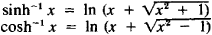### REFERENCE

Ianpol’skii, A. R. Giperbolicheskie funktsii. Moscow, 1960.
References in periodicals archive ?
However, Mazur's hyperbolic function and the AUC are arguably the two most commonly used measures of DD.
Finally, the contribution of this research will be the application of the life-cycle model, with both exponential and hyperbolic functions, to model the behavior of individuals affiliated to the Peruvian private pension system and its adaptation to the Peruvian situation.
Laibson (1997) used a quasi hyperbolic function to simplify the algorithm, because it can be expressed as a discrete time function.
The hyperbolic function is a general function whose special cases include the one-hoss-shay and straight-line cases.
Figure 1 shows the hyperbolic functions fitted to the median normalized indifference points estimated for each reward by all participants that were exposed to the seven delays.
The quantitative relation between the subjective value of the rewards and their delay of delivery is described by a hyperbolic function (Mazur, 1987), as follows:
The calculus preview in each chapter focuses on a single calculus concept, such as algebra and limits, the are problem and the hyperbolic functions.
The solid and dashed curves show the best-fitting hyperbolic functions (Equation 2) for the American and Japanese students, respectively.
Although hyperbolic functions are functions for a continuous variable, not for a discrete variable such as the number of beneficiaries, a function for a discrete variable can be approximated by the hyperbolic discount function (Equation 2) as shown by the present results and those of Jones and Rachlin (see Batschelet, 1979).
Hyperbolic functions accounted for more of the variance in both the reward and the cost condition, and delayed reward and cost discounting was significantly correlated.
Moreover, although the evidence is clear that delayed rewards are discounted according to hyperbolic function, there currently is no such clarity regarding discounting of delayed costs.

Site: Follow: Share:
Open / Close